## Bradut Apostol: Asymptotic properties of some functions related to regular integers modulo n, 221-231

### Abstract:

Let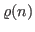denote the number of positive regular integers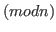less than or equal to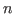and let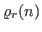(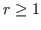) be the multidimensional generalization of the arithmetic function. We study the behaviour of the sequence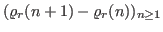. We also investigate the average orders of the functions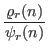,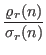and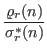. Here the functions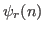,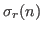,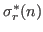generalize the Dedekind function, the sum of the divisors ofand the sum of the unitary divisors of, respectively. Finally, we give the extremal orders of some compositions involving the functions mentioned previously and the functions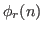and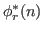which generalize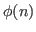, the Euler function and the unitary function corresponding to.

Key Words: arithmetical function, composition, regular integers, average orders, extremal orders.

2010 Mathematics Subject Classification: Primary 11A25,
Secondary 11N37